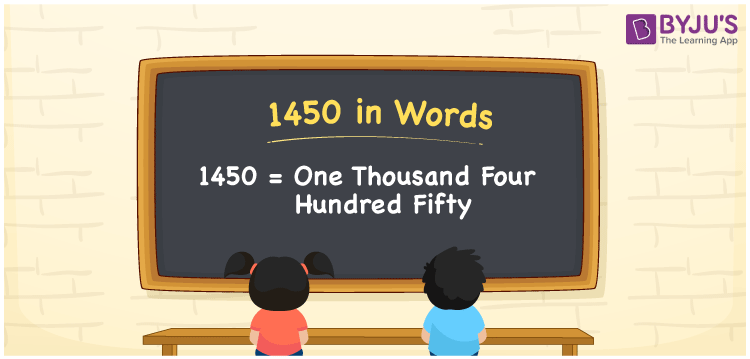# 1450 in Words

In English words, the number 1450 represents “One Thousand Four Hundred Fifty“. Knowing the numeral system can make converting numbers to words simple. It means that using the place value system to write 1450 in words is really beneficial. If the price of the cycle is Rs. 1450, the same can be written as “The price of the cycle is Rs. One thousand four hundred fifty”.Now let’s look at how to spell 1450 in English words with a detailed explanation.

 1450 in Words: One Thousand Four Hundred Fifty. One Thousand Four Hundred Fifty in Numerical Form: 1450.

## 1450 in English Words## How to Write 1450 in Words?

We can write 1450 in words using the place value table. The place value table for the number 1450 is shown below.

 Thousands Hundreds Tens Ones 1 4 5 0

The expanded form for the number 1450 is as follows:

= 1 × Thousand + 5 × Hundred + 5 × Ten + 0 × One

= 1 × 1000 + 4 × 100 + 5 × 10

= 1000 + 400 + 50

= 1450

= one thousand four hundred fifty

Hence, 1450 in words is one thousand four hundred fifty.

1450 in words – One thousand four hundred fifty

Is 1450 an odd number? – No

Is 1450 an even number? – Yes

Is 1450 a perfect square number? – No

Is 1450 a perfect cube number? – No

Is 1450 a prime number? – No

Is 1450 a composite number? – Yes

## Frequently Asked Questions on 1450 in Words

### How to write 1450 in words?

1450 in words is one thousand four hundred fifty.

### Simplify 1000 + 450, and express it in words.

Simplifying 1000 + 450, we get 1450. Hence, 1450 in words is one thousand four hundred fifty.

### Convert one thousand four hundred fifty into numbers.

One thousand four hundred fifty in numbers is 1450.